# Physics - Classical Mechanics - Exercises around Angular motion
[Image 1]

## Introduction

Hey it's a me again @drifter1! Today we continue with Physics and more specifically the branch "Classical Mechanics" to get into Exercises around Angular motion. I suggest you to read the previous articles of this chapter before this article. As always I will split the Examples into sections that correspond to the various articles of this chapter. So, without further ado, let's dive straight into it!

## Basics

Let's consider an object is moving along a circle with radius r = 0.5 m, needing T= 5 sec to do a complete cycle.

Calculate the following:
1. the angular velocity ω if it's angular acceleration is zero
2. the angular position θ after 8 seconds

### 1. Angular velocity

The circumference of a circle is given by 2πr.

Knowing that the object's period is 5 seconds and that it travels a distance of 2πr doing that cycle, we can easily calculate the average velocity of the object as: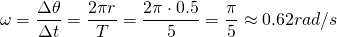### 2. Angular position

Using the same equation we can also calculate the position of the object after 8 seconds by simply substituting the calculated angular velocity ω and time, as following: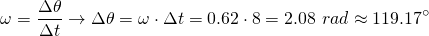## Constant Angular Acceleration

Let's now suppose an object that is moving in a circle of radius r = 5m with a constant angular acceleration of unknown value, starting at rest from θ = 0° and reaching an angular velocity ω = 30 rad/s after t = 15 sec.

Calculate the following:
1. The angular acceleration of the object
2. The angular position and velocity after t = 25 sec (from rest)

### 1. Angular acceleration

Angular velocity is given by the following equation that contains the initial and final angular velocity value, time and angular acceleration:
Knowing the time that has passed (t = 15 sec), the initial value (0 rad/s - at rest) and final value (30 rad/s - given) of angular velocity, we can easily find the angular acceleration: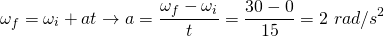### 2. Angular position and velocity

Using the same equation we can easily find the angular velocity after t = 25 seconds: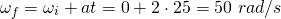Knowing that we start from θ = 0° degrees, we just have to substitute all our known quantities into the equation of angular position: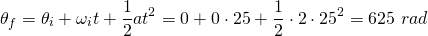## Rotational Kinetic Energy - Moment of Inertia

Suppose that we have a linear rod of length L = 0.4m and mass M = 1Kg. It has been proven that the Moment of Inertia about the center is given by: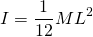Considering a constant angular velocity of ω = 1 rad/s, calculate :
1. The kinetic energy if the rod rotates about the center
2. The kinetic energy if the rod rotates about a point parallel to the center with a distance of d = 0.1m

### 1. Rotate about the center

To calculate the kinetic energy we just have to substitute the values into the equation of kinetic energy, as the moment of inertia about the center is already known: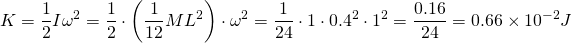### 2. Rotate about a point parallel to the center

When rotating about an axis parallel to an axis for which we know the moment of inertia, we have to use the Parallel Axis or Huygens-Steiner Theorem to find the moment of inertia along the parallel axis. That way we have:## Torque and Angular Acceleration

Let's consider a constant force F = 10N acts at an angle of θ = 45° on the second point (parallel to the center at a distance of d = 0.1m) of the previous example's object

Calculate:
1. The torque from that force
2. The angular acceleration of the object

### 1. Torque

Using the definition of torque we have: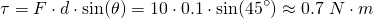### 2. Angular acceleration

The total torque is equal to the product of I and a, and thus using that we can calculate the angular acceleration, because the other quantities are known: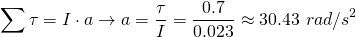## Rolling motion

Consider the same object as in the example about rotational kinetic energy. Let's suppose that this object also moves in Translational motion, meaning that it's axis of rotation is moving with some speed through space and time. Combining these two motions, we basically end up with Rolling motion. So, knowing that the speed of it's center of mass is v = 0.5 m/s and talking about the first case of rotating about the center, calculate the total kinetic energy of the object.

### Solution

The total kinetic energy is equal to the sum of rotational and translational kinetic energy. We already know the first term and so by simply calculating the translational kinetic energy, we can calculate the total energy as: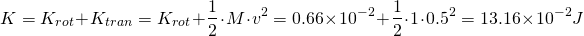## Work and Power

Let's calculate the work and power of the torque that we had in the example about torque, supposing a distance of 2π and that the rod starts at rest.

The angular velocity after travelling a distance of 2π is:### Work

From the Work-Energy Theorem, we know that the Work is equal to the change in kinetic energy. Because the initial kinetic energy is zero, we can easily calculate the work: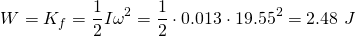### Power

We already calculated the final angular velocity and thus the power: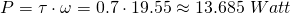## Angular Momentum

Let's lastly also calculate the angular momentum at the same moment as in the previous example (word play), as we already know all terms: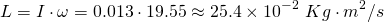## RESOURCES:

### References

My previous articles...

### Images

1.## Final words | Next up

And this is actually it for today's post!

Next time we will start a new Mechanics "chapter"...

See ya!Keep on drifting!

H2
H3
H4
3 columns
2 columns
1 column